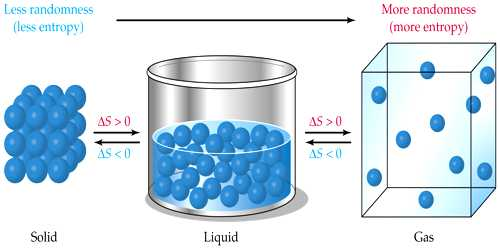# Significance of Entropy

Significance of Entropy

The thermal property that remains constant in an adiabatic process is called entropy. Alternately, entropy is the physical property of the body that remains constant in an adiabatic process. Entropy is a measure of the part that cannot be used to do work. So entropy is useful in that it provides the information regarding structural changes accompanying a given process. In a way, entropy is useful for knowing the efficiency of the internal energy of something. Hence, there is a close relationship between entropy S and the thermodynamic probability W of the state of the system. This relationship is expressed by Boltzmann as:

S = k ln W + constant

Where k denotes the Boltzmann constant which is equal to gas constant per single molecule i.e. R/NA.

Entropy is very significant in thermodynamics. The significances are mentioned below:

• Entropy is a physical quantity. It is equal to the ratio of heat absorbed or rejected to the temperature.
• It indicates the direction of heat flow. A spontaneous process is also known as an irreversible process because these processes take place at a very fast rate. All the natural processes are spontaneous processes.
• It helps in determining the thermodynamic state of an object. A little consideration will show that when a spontaneous process takes place it moves from less probable state to a more probable state.
• Like temperature, pressure, volume, internal energy, magnetic behavior it expresses the state of a body.
• The orderliness of an object decreases with the increase of entropy. Hence spontaneous processes are accompanied by an increase in entropy as well as an increase in the disorder of the system.
• Like temperature or pressure, it cannot be felt. Hence the state of the order of the molecules gets disturbed and gives rise to greater disorder.
• It is a measure of the disorder or randomness in the system. When a gas expands into a vacuum, water flows out of a reservoir, spontaneous chain reaction takes place, an increase in the disorder occurs and therefore entropy increases.

Entropy is the measure of the disorder or randomness in a system. The entropy of a substance is a real physical quantity and is a definite function of the state of the body like pressure, temperature, the volume of internal energy. When a substance is heated or cooled there is also a change in entropy. Thus increase in entropy implies a transition from one ordered to a less ordered state of affair. In other words, if you drop a box of matches on the floor, the matches will fall all over the place, which is an increase in entropy.

Entropy is a ‘measure of disorder’ of a system. A ‘measure of unavailable energy’.

Entropy level higher in Gas: Solid< Liquid< Gas.